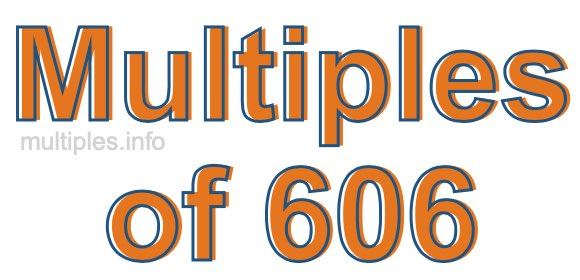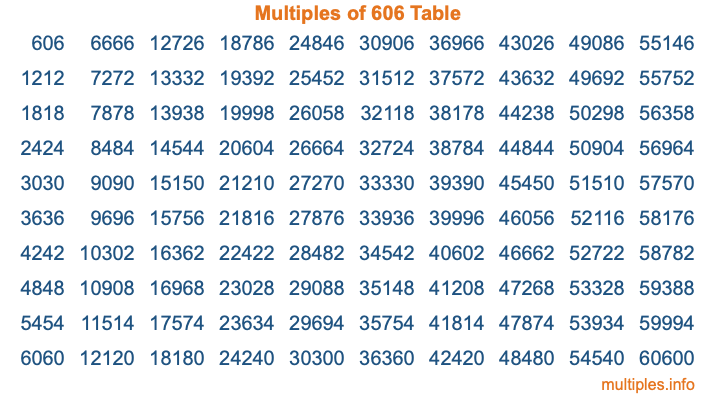Multiples of 606Welcome to the Multiples of 606 page. Here we will first teach you everything you will ever need to know about the multiples of 606, and then give you a study guide summary of everything we taught you to make sure you remember it all. Use this page to look up facts and learn information about the multiples of 606. This page will make you a multiples of six hundred six expert!

Definition of Multiples of 606
Multiples of 606 are all the numbers that when divided by 606 equal an integer. Each of the multiples of 606 are called a multiple. A multiple of 606 is created by multiplying 606 by an integer.

Therefore, to create a list of multiples of 606, you start with 1 multiplied by 606, then 2 multiplied by 606, then 3 multiplied by 606, and so on for as long as you want. Thus, the list of the first five multiples of 606 is 606, 1212, 1818, 2424, and 3030. To see a larger list of multiples of 606, see the printable image of Multiples of 606 further down on this page. We also have a category where you can choose any nth multiple of 606.

Multiples of 606 Checker
The Multiples of 606 Checker below checks to see if any number of your choice is a multiple of 606. In other words, it checks to see if there is any number (integer) that when multiplied by 606 will equal your number. To do that, we divide your number by 606. If the the quotient is an integer, then your number is a multiple of 606.

Is  a multiple of 606?

Least Common Multiple of 606 and ...
A Least Common Multiple (LCM) is the lowest multiple that two or more numbers have in common. This is also called the smallest common multiple or lowest common multiple and is useful to know when you are adding our subtracting fractions. Enter one or more numbers below (606 is already entered) to find the LCM.

Check out our LCM Calculator if you need more details about the Least Common Multiple or if you need the LCM for different numbers for adding and subtraction fractions.

nth Multiple of 606
As we stated above, 606 is the first multiple of 606, 1212 is the second multiple of 606, 1818 is the third multiple of 606, and so on. Enter a number below to find the nth multiple of 606.

th multiple of 606

Multiples of 606 vs Factors of 606
606 is a multiple of 606 and a factor of 606, but that is where the similarities end. All postive multiples of 606 are 606 or greater than 606. All positive factors of 606 are 606 or less than 606.

Below is the beginning list of multiples of 606 and the factors of 606 so you can compare:

Multiples of 606: 606, 1212, 1818, 2424, 3030, etc.

Factors of 606: 1, 2, 3, 6, 101, 202, 303, 606

As you can see, the multiples of 606 are all the numbers that you can divide by 606 to get a whole number. The factors of 606, on the other hand, are all the whole numbers that you can multiply by another whole number to get 606.

It's also interesting to note that if a number (x) is a factor of 606, then 606 will also be a multiple of that number (x).

Multiples of 606 vs Divisors of 606
The divisors of 606 are all the integers that 606 can be divided by evenly. Below is a list of the divisors of 606.

Divisors of 606: 1, 2, 3, 6, 101, 202, 303, 606

The interesting thing to note here is that if you take any multiple of 606 and divide it by a divisor of 606, you will see that the quotient is an integer.

Multiples of 606 Table
Below is an image of the first 100 multiples of 606 in a table. The table is in chronological order, column by column. The first column has the first ten multiples of 606, the second column has the next ten multiples of 606, and so on.The Multiples of 606 Table is also referred to as the 606 Times Table or Times Table of 606. You are welcome to print out our table for your studies.

Negative Multiples of 606
Although not often discussed or needed in math, it is worth mentioning that you can make a list of negative multiples of 606 by multiplying 606 by -1, then by -2, then by -3, and so on, to get the following list of negative multiples of 606:

-606, -1212, -1818, -2424, -3030, etc.

Multiples of 606 Summary
Below is a summary of important Multiples of 606 facts that we have discussed on this page. To retain the knowledge on this page, we recommend that you read through the summary and explain to yourself or a study partner why they hold true.

There are an infinite number of multiples of 606.

A multiple of 606 divided by 606 will equal a whole number.

606 divided by a factor of 606 equals a divisor of 606.

The nth multiple of 606 is n times 606.

The largest factor of 606 is equal to the first positive multiple of 606.

606 is a multiple of every factor of 606.

606 is a multiple of 606.

A multiple of 606 divided by a divisor of 606 equals an integer.

606 divided by a divisor of 606 equals a factor of 606.

Any integer times 606 will equal a multiple of 606.

Multiples of a Number
Here you can get the multiples of another number, all with the same attention to detail as we did for multiples of 606 on this page.

Multiples of
Multiples of 607
Did you find our page about multiples of six hundred six educational? Do you want more knowledge? Check out the multiples of the next number on our list!